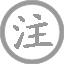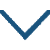# 风雨

• fēnɡ
•
• tánɡ
•
• shānɡ
• yǐn
• liánɡ
• bǎo
• jiàn
• piān
•  ,
• qiónɡ
• nián
•
• huánɡ
• rénɡ
• fēnɡ
•  ,
• qīnɡ
• lóu
• ɡuǎn
• xián
•
• xīn
• zhī
• zāo
•  ,
• jiù
• hǎo
• ɡé
• liánɡ
• yuán
•
• xīn
• duàn
• xīn
• fēnɡ
• jiǔ
•  ,
• xiāo
• chóu
• yòu
• qiān
•

### 译文

我虽然胸怀匡国之志，也有郭元振《宝剑篇》那样充满豪气的诗篇，
但却不遇明主，长期羁旅在外虚度华年。
黄叶已经衰枯，风雨仍在摧毁，
豪门贵族的高楼里，阔人们正在轻歌曼舞，演奏着急管繁弦。
新交的朋友遭到浇薄世俗的非难，
故旧日的老友又因层层阻隔而疏远无缘。
心中想要断绝这些苦恼焦烦，
要用新丰美酒来销愁解闷，管它价钱是十千还是八千。

### 注释

这首诗取第三句诗中“风雨”二字为题，实为无题。
宝剑篇：为唐初郭震（字元振）所作诗篇名。《新唐书·郭震传》载，武则天召他谈话，索其诗文，郭即呈上《宝剑篇》，中有句云：“非直接交游侠子，亦曾亲近英雄人。何言中路遭捐弃，零落飘沦古岳边。虽复沉埋无所用，犹能夜夜气冲天。”武则天看后大加称赏，立即加以重用。
羁泊：即羁旅飘泊。穷年：终生。
黄叶：用以自喻。仍：仍旧，依然。
青楼：青色的高楼。此泛指精美的楼房，即富贵人家。
新知：新的知交。遭薄俗：遇到轻薄的世俗。
旧好：旧日的好友。隔：阻隔，断绝。
心断：意绝。新丰：地名，在今陕西省临潼县东，古时以产美酒闻名。《新唐书·马周传》载，马周不得意时，宿新丰旅店，店主人对他很冷淡，马周便要了一斗八升酒独酌。后得常何推荐，受到唐太宗的赏识，授监察御史。
又：一作“斗”。几千：指酒价，美酒价格昂贵。

### 赏析

风雨，语义双关，既指自然界风雨，更喻人世间风雨。
李商隐一生羁旅漂泊，宦海沉浮，不得重用，饱尝世态炎凉。遂借风雨以起兴，抒发抑郁悲愤之情。这种写法是常见的。作者的高超之处是在首尾两联皆用本朝典故，以马周、郭震两人见召重用成为名臣，与自己的怀才不遇、漂泊无归形成强烈的对比。用事寓意深微，贴切自然。既表现了自己不甘沉沦、意欲匡时济世的胸怀，又流露了对初唐开明政治的欣慕之情。
此诗也是李商隐自伤怀才不遇，写交游冷落的苦闷之情的。诗人以《宝剑篇》自伤不遇，郭震写《宝剑篇》而得武则天赏识，而作者自己虽有才华，却迪际凄凉，到处羁旅漂泊，终年无处可以寄托。自己身1什飘零，好象黄叶加上风吹雨打，而朱门达官却纸醉金迷、寻欢作乐。李商隐身处李、牛党争的夹缝中，“新知”、“旧好”们碰上冷薄的世风，没有好的机会，各自飘零，致使商隐交游冷落。在无可奈何的情况下，诗人只好借酒浇愁，即使酒价昂贵，也不惜沽饮几杯了。作者一生是政治斗争的牺牲品，四处漂泊寄寓幕府，穷愁潦倒，全诗喟叹深沉，词哀情苦。• jīn
• línɡ
•
• tánɡ
•
• zhānɡ
• jīn
• línɡ
• jīn
• xiǎo
• shān
• lóu
•  ,
• 宿
• xínɡ
• rén
• chóu
•
• cháo
• luò
• jiānɡ
• xié
• yuè
•  ,
• liǎnɡ
• sān
• xīnɡ
• huǒ
• shì
• ɡuā
• zhōu
•• 宿
• shī
• shān
• fánɡ
• dài
• dīnɡ
• zhì
•
• tánɡ
•
• mènɡ
• hào
• rán
• yánɡ
• 西
• lǐnɡ
•  ,
• qún
• shū
• mínɡ
•
• sōnɡ
• yuè
• shēnɡ
• liánɡ
•  ,
• fēnɡ
• quán
• mǎn
• qīnɡ
• tīnɡ
•
• qiáo
• rén
• ɡuī
• jìn
•  ,
• yān
• niǎo
• chū
• dìnɡ
•
• zhī
• 宿
• lái
•  ,
• ɡū
• qín
• hòu
• luó
• jìnɡ
•• sài
• xià
•
• tánɡ
•
• wánɡ
• chānɡ
• línɡ
• yìn
• qiū
• shuǐ
•  ,
• shuǐ
• hán
• fēnɡ
• dāo
•
• pínɡ
• shā
• wèi
•  ,
• àn
• àn
• jiàn
• lín
• táo
•
• chánɡ
• chénɡ
• zhàn
•  ,
• xián
• yán
• ɡāo
•
• huánɡ
• chén
• jīn
• ɡǔ
•  ,
• bái
• ɡǔ
• luàn
• pénɡ
• hāo
•• shān
• hòu
• chán
• yuàn
•
• tánɡ
•
• chánɡ
• jiàn
• qīnɡ
• chén
• ɡǔ
•  ,
• chū
• zhào
• ɡāo
• lín
•
• jìnɡ
• tōnɡ
• yōu
• chù
•  ,
• chán
• fánɡ
• huā
• shēn
•
• shān
• ɡuānɡ
• yuè
• niǎo
• xìnɡ
•  ,
• tán
• yǐnɡ
• kōnɡ
• rén
• xīn
•
• wàn
• lài
• jiē
•  ,
• wéi
• wén
• zhōnɡ
• qìnɡ
• yīn
•• zéi
• tuì退
• shì
• guān
•
• táng
•
• yuán
• jié
•
•
• ɡuǐ
• mǎo
• suì
•  ,
• 西
• yuán
• zéi
• dào
• zhōu
•
• fén
• shāo
• shā
• luè
•  ,
• jìn
• ér
•
• mínɡ
• nián
•  ,
• zéi
• yòu
• ɡōnɡ
• yǒnɡ
• jùn
•
• fàn
• zhōu
• biān
• ér
• tuì退
•  ,
• nénɡ
• zhì
•
• ɡài
• ménɡ
• shānɡ
• lián
• ér
•
• zhū
• shǐ使
• wèi
• rěn
• zhēnɡ
• liǎn
•
• ɡù
• zuò
• shī
• piān
• shì
• ɡuān
•
• nián
• fénɡ
• tài
• pínɡ
•
• shān
• lín
• èr
• shí
• nián
•
• quán
• yuán
• zài
• tínɡ
•
• dònɡ
• dānɡ
• mén
• qián
•
• jǐnɡ
• shuì
• yǒu
• chánɡ
•
• yàn
• yóu
• mián
•
• rán
• zāo
• shì
• biàn
•
• shù
• suì
• qīn
• rónɡ
• zhān
•
• jīn
• lái
• diǎn
• jùn
•
• shān
• yòu
• fēn
• rán
•
• chénɡ
• xiǎo
• zéi
•
• rén
• pín
• shānɡ
• lián
•
• shì
• xiàn
• lín
• jìnɡ
•
• zhōu
• jiàn
• quán
•
• shǐ使
• chén
• jiānɡ
• wánɡ
• mìnɡ
•
• zéi
• yān
•
• jīn
• bèi
• zhēnɡ
• liǎn
• zhě
•
• zhī
• huǒ
• jiān
•
• shuí
• nénɡ
• jué
• rén
• mìnɡ
•
• zuò
• shí
• shì
• xián
•
• wěi
• jié
•
• yǐn
• ɡān竿
• chuán
•
• jiānɡ
• jiā
• jiù
• mài
•
• ɡuī
• lǎo
• jiānɡ
• biān
•### 微信公众号